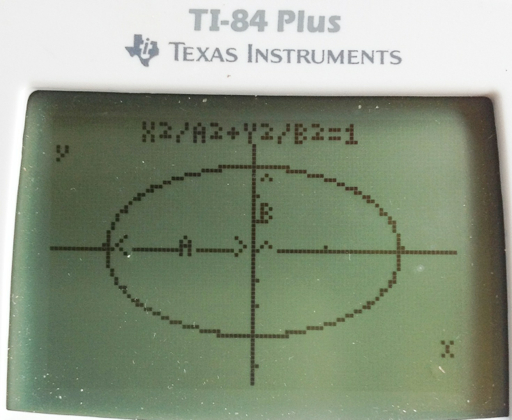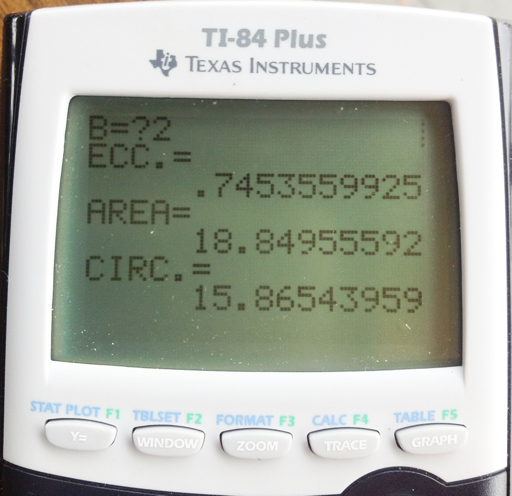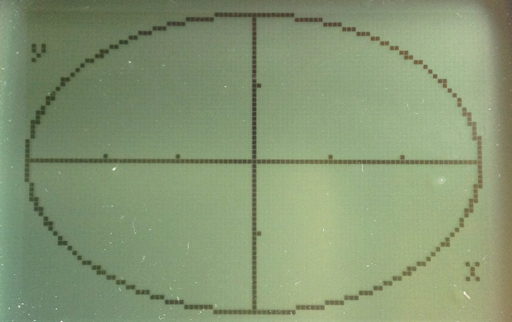## Saturday, August 4, 2012

### Applications and Programming: Ellipses

The following program works with several properties with ellipses. The general equation for an ellipse with center (x0, y0) is:

(x - x0)^2 / a^2 + (y -y0)^2 / b^2 = 1

For today's blog, I will center the ellipse at the origin (0,0).With a and b as the length of the semi-axes:

* min(a,b) is the length of the semi-minor axis. min is the Minimum function, the least of a and b.
* max(a,b) is the length of the semi-major axis. max is the Maximum function, the greater of a and b.
* The eccentricity of the ellipse is e = √ (1 - [min(a,b) / max(a,b)]^2). In this sense, e is not the constant 2.7182818285... . If e = 0, then the ellipse is actually a circle. Further more, you can determine where the foci are, which is the distance e * max(a,b) along the semi-major axis.
* The area of an ellipse is easy enough: A = π a b
* The circumference is a different story. A good estimate, suggest by the NCEES, if a and b are close together is C ≈ 2 π √ ( [a^2 - b^2] / 2)

Finding the true circumference will require some calculus. Let the ellipse be defined by the parametric equations:

x(t) = a cos t
y(t) = b sin t
With 0 ≤ t ≤ 2 π

The arc length of a parametric equation is

∫ ( √ ( (dx/dt)^2 + (dy/dt)^2 ) dt, t0, t1)

Which means that the true circumference of an ellipse is

∫ ( √ ( a^2 sin(t)^2 + b^2 cos(t)^2 ) dt, 0, 2 π )

Any calculator with integration can handle this.

The ELLIPSE Program, TI-84+, 184 bytes

The following program will:
1. Prompt for a and b. (A, B)
2. Calculate eccentricity (E).
3. Calculate area (A).
4. Calculate circumference (C).
5. Draw the ellipse. The program leaves the user on the graph screen. The ellipse can be traced and used for further analysis if desired.

ELLIPSE - TI-84+

: Param
: Disp "X^2/A^2+Y^2/B^2 =1"
: Prompt A,B
: "A cos(T)"→X1T
: "B sin(T)" →Y1T
: FnOff
: PlotsOff
: FnOn 1
: 0 →Tmin
: 2 π →Tmax
: π / 128 →Tstep
: √(1-(min(A,B)/max(A,B))² → E
: π A B → R
: fnInt(√(A² sin(T)² + B² cos(T)² , T, 0, 2 π) → C
: Disp "ECC.=", E
: Pause
: Disp "AREA=", R
: Pause
: Disp "CIRC.=",C
: Pause
: ZoomFit

Here is the ELLIPSE program executed with A=2 and B=3:I thank you once again. Thank you to the followers of my blog, love the comments, I appreciate it.

Until next time,

Eddie

This blog is property of Edward Shore. © 2012

#### 1 comment:

1.This comment has been removed by the author.

### Retro Review: Radio Shack EC-2024

Retro Review:  Radio Shack EC-2024 Quick Facts Company:  Radio Shack Years in Production: Around 1994 Original Price: \$24.99 Bat...